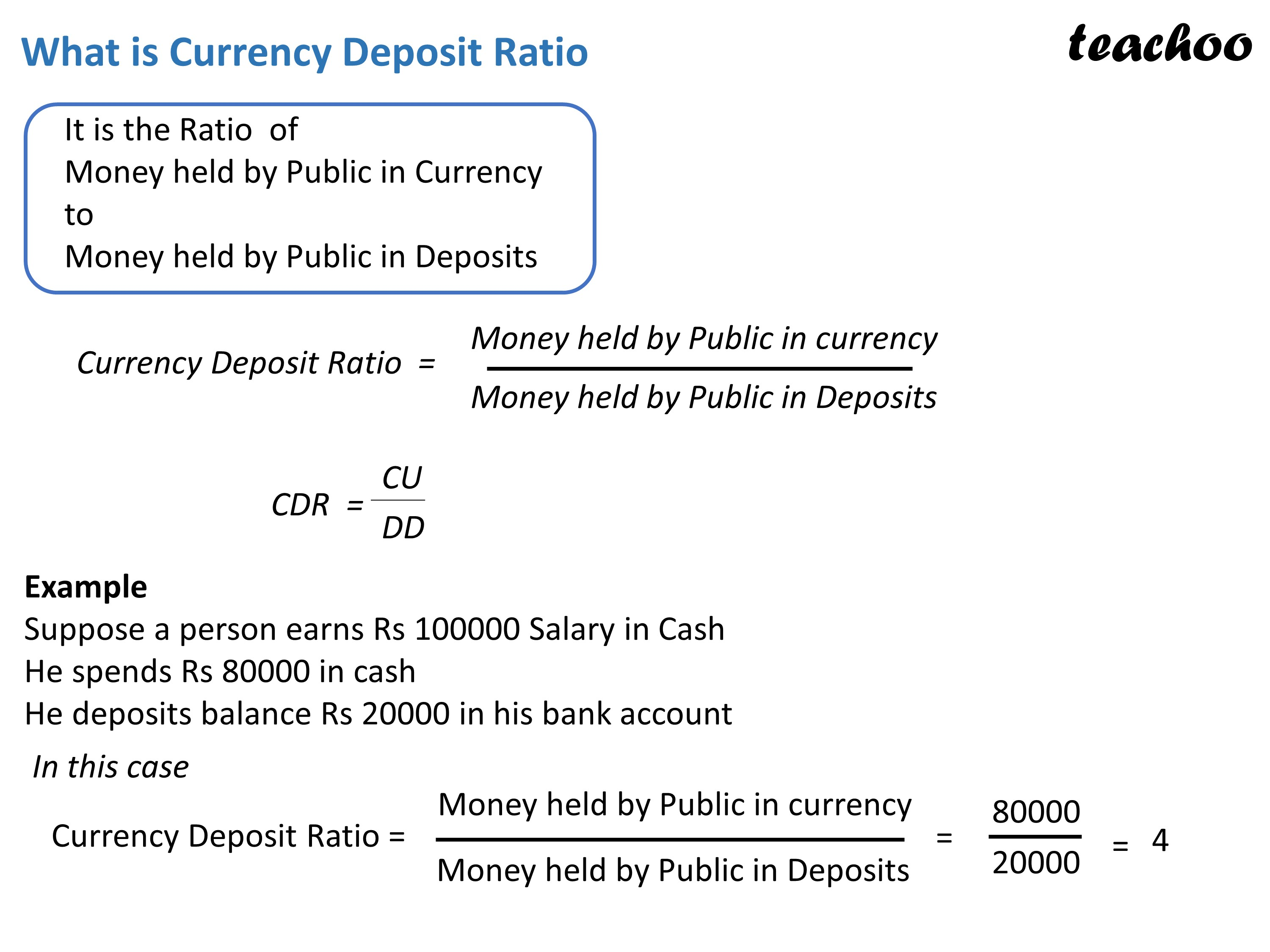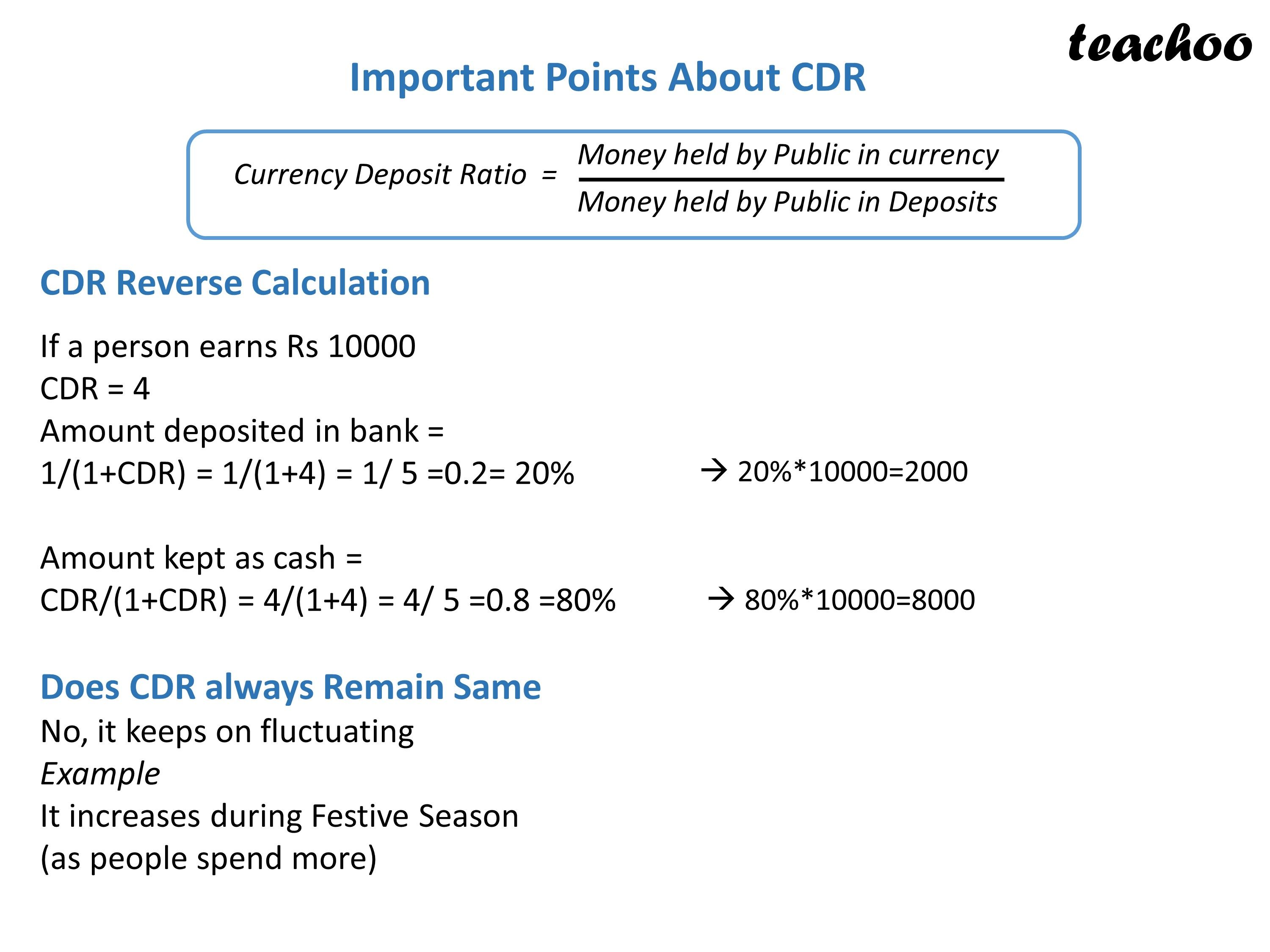Chapter 3 Part 2 - Banking

Economics Class 12
Macroeconomics

Currency Deposit Ratio - Meaning, Importance and CalculationGet live Maths 1-on-1 Classs - Class 6 to 12

### Transcript

What is Currency Deposit Ratio It is the Ratio of Money held by Public in Currency to Money held by Public in Deposits Currency Deposit Ratio = Money held by Public in currency Money held by Public in Deposits Example Suppose a person earns Rs 100000 Salary in Cash He spends Rs 80000 in cash He deposits balance Rs 20000 in his bank account In this case Currency Deposit Ratio = Money held by Public in Deposits Money held by Public in currency Important Points About CDR CDR Reverse Calculation If a person earns Rs 10000 CDR = 4 Amount deposited in bank = 1/(1+CDR) = 1/(1+4) = 1/ 5 =0.2= 20% Amount kept as cash = CDR/(1+CDR) = 4/(1+4) = 4/ 5 =0.8 =80% Does CDR always Remain Same No, it keeps on fluctuating Example It increases during Festive Season (as people spend more)